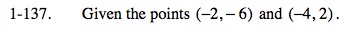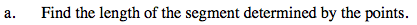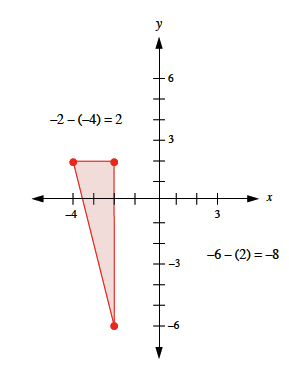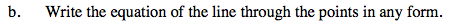### Home > PC > Chapter 1 > Lesson 1.4.1 > Problem1-137

1-137.Use the distance formula if you know it, or sketch the points, make a right triangle, and use the Pythagorean theorem.$\text{distance}=\sqrt{2^2 + (-8)^2}\ \ \footnotesize =\sqrt{4 + 64}\ \ \footnotesize = \sqrt{68}$$\text{Use:\ }m= \frac{\text{rise}}{\text{run}} \\ \ \ \ \ \ \ \ \ \ \ \footnotesize \frac{y_2 - y_1}{x_2 - x_1}$